# Division

Divide by the number 0.2 is the same as multiply by what number?

Correct result:

x =  5

#### Solution:

$x=1\mathrm{/}0.2=5$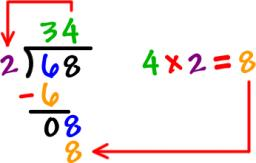We would be pleased if you find an error in the word problem, spelling mistakes, or inaccuracies and send it to us. Thank you!## Next similar math problems:

• BicycleThe bicycle pedal gear has 36 teeth, the rear gear wheel has 10 teeth. How many times turns rear wheel, when pedal wheel turns 120x?
• Expanded formWhat is the expanded form of 0.21?
• Reciprocal equation 3Solve reciprocal equation: 1/2 + 2/3=1/x
• MidpointsTriangle whose sides are midpoints of sides of triangle ABC has a perimeter 45. How long is perimeter of triangle ABC?
• Soccer team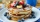Hilahs soccer team is trying to raise $2414 to travel to a tournament in Florida, so they decided to host a pancake for the breakfast. How many people need to attend their breakfast in order to raise$2414, if profit per one pancake is \$1.5?
• Without 2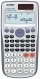Without multipying, tell whether the product 0.644 x 0.25 will be greater than 1 or less than 1? Explane how you know. Then find the product.
• A numberA number increased by 7.9 is 8.3
• The ketchup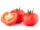If 3 1/4 of tomatoes are needed to make 1 bottle of ketchup. Find the number of tomatoes required to make 4 1/5 bottles
• Simplify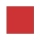Simplify powers multiplication: (3+22)(5-42)
• Quotient 3If the quotient of 8/13 and 2 is subtracted from the product of 1 3/4 and 8/21, what is the difference?
• Promile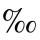Calculate the 4.6 ‰ of 199.
• Bottles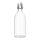The weight of 17 bottles is 91.7 kg. What is the weight of 1 bottle?
• Zhiwei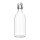Zhiwei wants to divide 19 litres of water equally into 6 pots. Find the volume of water in each pot?
• Double 5Peter was thinking of a number. Peter doubles it and gets an answer of 8.6. What was the original number?
• EvaluateThe division of numbers 18 and 6 increase by product of the numbers 156 and 0.001
• SymbolsIf 2*3 = 60 ; 3*4 = 120 and 4*5 = 200, what is 2*5?
• Numbers divisionWith what number should be divided mixed number 2 3/4 to get 11/12?